## What is the distance between (-6,0) and (5,3)?

Question

What is the distance between (-6,0) and (5,3)?

in progress 0
6 months 2021-08-24T03:56:56+00:00 1 Answers 0 views 0

## Answers ( )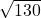or 11.4

Step-by-step explanation:

Use the distance formula:

d =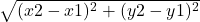Plug in the points:

d =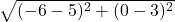Simplify:

d =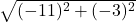d =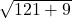d == approximately 11.4

So, the distance isor approx. 11.4